### Infinite body with a circular hole, steady 1-D.

R10 Infinite body surrounding a circular hole, a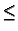r <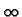, with G = 0 (Dirichlet) at r = a.

2GR10(r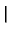r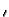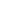) =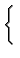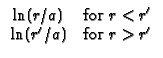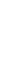R20 Infinite body surrounding a circular hole, ar <, with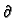G/r = 0 (Neumann) at r = a. Note that this geometry requires a pseudo GF, denoted H. The temperature solution found from a pseudo GF requires that the total volumetric heat flow is equal to the boundary heat flow, and the spatial average temperature in the body must be supplied as a known condition.

2HR20(rr) =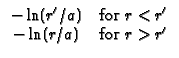R30 Infinite body surrounding a circular hole, ar <, with - kG/r + h1G = 0 (convection) at r = a. Note: B1 = h1a/k.

2GR30(rr) =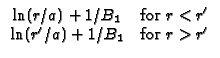Kevin D. Cole
2002-12-31